# modelAccuracyPlot

Scatter plot of predicted and observed LGDs

## Syntax

``modelAccuracyPlot(lgdModel,data)``
``modelAccuracyPlot(___,Name,Value)``
``h = modelAccuracyPlot(ax,___,Name,Value)``

## Description

example

````modelAccuracyPlot(lgdModel,data)` returns a scatter plot of observed vs. predicted loss given default (LGD) data with a linear fit. `modelAccuracyPlot` supports comparison against a reference model. By default, `modelAccuracyPlot` plots in the LGD scale. ```

example

````modelAccuracyPlot(___,Name,Value)` specifies options using one or more name-value pair arguments in addition to the input arguments in the previous syntax. You can use the `ModelLevel` name-value pair argument to compute metrics using the underlying model's transformed scale. ```

example

````h = modelAccuracyPlot(ax,___,Name,Value)` specifies options using one or more name-value pair arguments in addition to the input arguments in the previous syntax and returns the figure handle `h`.```

## Examples

collapse all

This example shows how to use `fitLGDModel` to fit data with a `Regression` model and then use `modelAccuracyPlot` to generate a scatter plot for predicted and observed LGDs.

Load the loss given default data.

```load LGDData.mat head(data)```
```ans=8×4 table LTV Age Type LGD _______ _______ ___________ _________ 0.89101 0.39716 residential 0.032659 0.70176 2.0939 residential 0.43564 0.72078 2.7948 residential 0.0064766 0.37013 1.237 residential 0.007947 0.36492 2.5818 residential 0 0.796 1.5957 residential 0.14572 0.60203 1.1599 residential 0.025688 0.92005 0.50253 investment 0.063182 ```

Partition Data

Separate the data into training and test partitions.

```rng('default'); % for reproducibility NumObs = height(data); c = cvpartition(NumObs,'HoldOut',0.4); TrainingInd = training(c); TestInd = test(c);```

Create `Regression` LGD Model

Use `fitLGDModel` to create a `Regression` model using training data.

```lgdModel = fitLGDModel(data(TrainingInd,:),'regression'); disp(lgdModel) ```
``` Regression with properties: ResponseTransform: "logit" BoundaryTolerance: 1.0000e-05 ModelID: "Regression" Description: "" UnderlyingModel: [1x1 classreg.regr.CompactLinearModel] PredictorVars: ["LTV" "Age" "Type"] ResponseVar: "LGD" ```

Display the underlying model.

`disp(lgdModel.UnderlyingModel)`
```Compact linear regression model: LGD_logit ~ 1 + LTV + Age + Type Estimated Coefficients: Estimate SE tStat pValue ________ ________ _______ __________ (Intercept) -4.7549 0.36041 -13.193 3.0997e-38 LTV 2.8565 0.41777 6.8377 1.0531e-11 Age -1.5397 0.085716 -17.963 3.3172e-67 Type_investment 1.4358 0.2475 5.8012 7.587e-09 Number of observations: 2093, Error degrees of freedom: 2089 Root Mean Squared Error: 4.24 R-squared: 0.206, Adjusted R-Squared: 0.205 F-statistic vs. constant model: 181, p-value = 2.42e-104 ```

Generate Scatter Plot of Predicted and Observed LGDs

Use `modelAccuracyPlot` to generate a scatter plot of predicted and observed LGDs for the test data set. The `ModelLevel` name-value pair argument modifies the output only for `Regression` models, not `Tobit` models, because there are no response transformations for the `Tobit` model.

`modelAccuracyPlot(lgdModel,data(TestInd,:),'ModelLevel',"underlying")`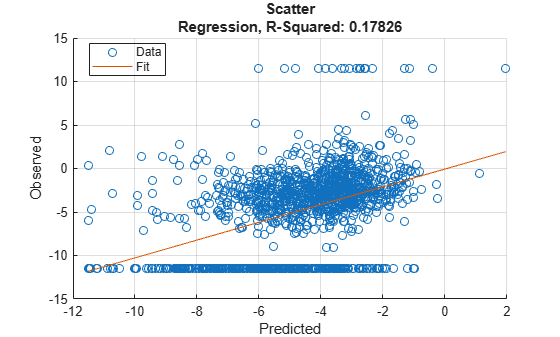This example shows how to use `fitLGDModel` to fit data with a `Tobit` model and then use `modelAccuracyPlot` to generate a scatter plot of predicted and observed LGDs.

Load the loss given default data.

```load LGDData.mat head(data)```
```ans=8×4 table LTV Age Type LGD _______ _______ ___________ _________ 0.89101 0.39716 residential 0.032659 0.70176 2.0939 residential 0.43564 0.72078 2.7948 residential 0.0064766 0.37013 1.237 residential 0.007947 0.36492 2.5818 residential 0 0.796 1.5957 residential 0.14572 0.60203 1.1599 residential 0.025688 0.92005 0.50253 investment 0.063182 ```

Partition Data

Separate the data into training and test partitions.

```rng('default'); % for reproducibility NumObs = height(data); c = cvpartition(NumObs,'HoldOut',0.4); TrainingInd = training(c); TestInd = test(c);```

Create Tobit LGD Model

Use `fitLGDModel` to create a `Tobit` model using training data.

```lgdModel = fitLGDModel(data(TrainingInd,:),'tobit'); disp(lgdModel) ```
``` Tobit with properties: CensoringSide: "both" LeftLimit: 0 RightLimit: 1 ModelID: "Tobit" Description: "" UnderlyingModel: [1x1 risk.internal.credit.TobitModel] PredictorVars: ["LTV" "Age" "Type"] ResponseVar: "LGD" ```

Display the underlying model.

`disp(lgdModel.UnderlyingModel)`
```Tobit regression model: LGD = max(0,min(Y*,1)) Y* ~ 1 + LTV + Age + Type Estimated coefficients: Estimate SE tStat pValue _________ _________ _______ __________ (Intercept) 0.058257 0.027265 2.1367 0.032737 LTV 0.20126 0.031354 6.4189 1.6932e-10 Age -0.095407 0.0072653 -13.132 0 Type_investment 0.10208 0.018058 5.6531 1.7915e-08 (Sigma) 0.29288 0.0057036 51.35 0 Number of observations: 2093 Number of left-censored observations: 547 Number of uncensored observations: 1521 Number of right-censored observations: 25 Log-likelihood: -698.383 ```

Generate Scatter Plot of Predicted and Observed LGDs

Use `modelAccuracyPlot` to generate a scatter plot of predicted and observed LGDs for the test data set.

`modelAccuracyPlot(lgdModel,data(TestInd,:))`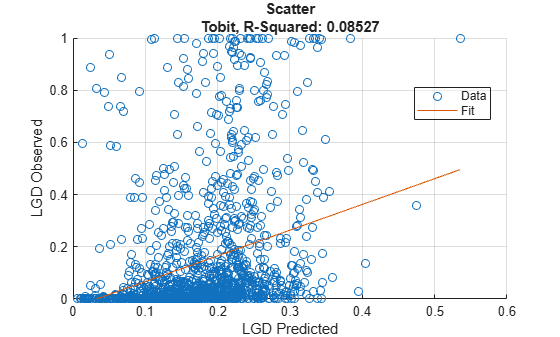`modelAccuracyPlot` generates a scatter plot of observed vs. predicted LGD values. The `'XData'` and `'YData'` name-value pair arguments allow you to visualize the residuals or generate a scatter plot against a variable of interest.

Load the loss given default data.

```load LGDData.mat head(data)```
```ans=8×4 table LTV Age Type LGD _______ _______ ___________ _________ 0.89101 0.39716 residential 0.032659 0.70176 2.0939 residential 0.43564 0.72078 2.7948 residential 0.0064766 0.37013 1.237 residential 0.007947 0.36492 2.5818 residential 0 0.796 1.5957 residential 0.14572 0.60203 1.1599 residential 0.025688 0.92005 0.50253 investment 0.063182 ```

Partition Data

Separate the data into training and test partitions.

```rng('default'); % for reproducibility NumObs = height(data); c = cvpartition(NumObs,'HoldOut',0.4); TrainingInd = training(c); TestInd = test(c);```

Create Regression LGD Model

Use `fitLGDModel` to create a `Regression` model using training data.

```lgdModel = fitLGDModel(data(TrainingInd,:),'regression'); disp(lgdModel)```
``` Regression with properties: ResponseTransform: "logit" BoundaryTolerance: 1.0000e-05 ModelID: "Regression" Description: "" UnderlyingModel: [1x1 classreg.regr.CompactLinearModel] PredictorVars: ["LTV" "Age" "Type"] ResponseVar: "LGD" ```

Display the underlying model.

`disp(lgdModel.UnderlyingModel)`
```Compact linear regression model: LGD_logit ~ 1 + LTV + Age + Type Estimated Coefficients: Estimate SE tStat pValue ________ ________ _______ __________ (Intercept) -4.7549 0.36041 -13.193 3.0997e-38 LTV 2.8565 0.41777 6.8377 1.0531e-11 Age -1.5397 0.085716 -17.963 3.3172e-67 Type_investment 1.4358 0.2475 5.8012 7.587e-09 Number of observations: 2093, Error degrees of freedom: 2089 Root Mean Squared Error: 4.24 R-squared: 0.206, Adjusted R-Squared: 0.205 F-statistic vs. constant model: 181, p-value = 2.42e-104 ```

Generate Scatter Plot of Predicted and Observed LGDs

Use `modelAccuracyPlot` to generate a scatter plot of residuals against LTV values.

`modelAccuracyPlot(lgdModel,data(TestInd,:),'XData','LTV','YData','residuals')`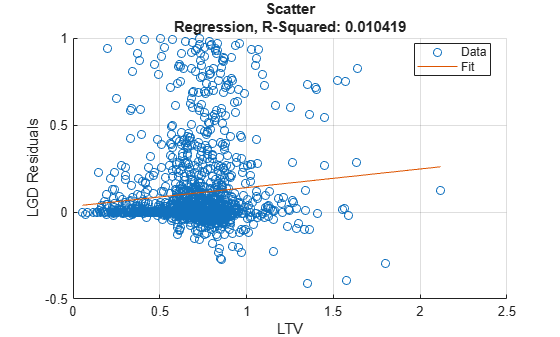For `Regression` models, the `'ModelLevel'` name-value pair argument allows you to visualize the plot using the underlying model scale.

`modelAccuracyPlot(lgdModel,data(TestInd,:),'XData','LTV','YData','residuals','ModelLevel','underlying')`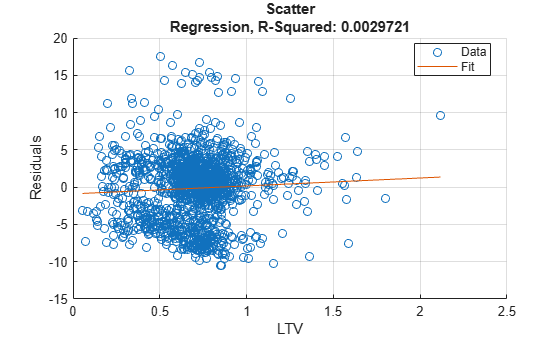For categorical variables, `modelAccuracyPlot` uses a swarm chart. For more information, see `swarmchart`.

`modelAccuracyPlot(lgdModel,data(TestInd,:),'XData','Type','YData','residuals','ModelLevel','underlying')`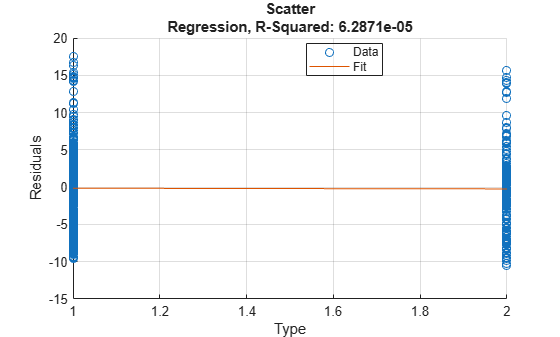## Input Arguments

collapse all

Loss given default model, specified as a previously created `Regression` or `Tobit` object using `fitLGDModel`.

Data Types: `object`

Data, specified as a `NumRows`-by-`NumCols` table with predictor and response values. The variable names and data types must be consistent with the underlying model.

Data Types: `table`

(Optional) Valid axis object, specified as an `ax` object that is created using `axes`. The plot will be created in the axes specified by the optional `ax` argument instead of in the current axes (gca). The optional argument `ax` must precede any of the input argument combinations.

Data Types: `object`

### Name-Value Pair Arguments

Specify optional comma-separated pairs of `Name,Value` arguments. `Name` is the argument name and `Value` is the corresponding value. `Name` must appear inside quotes. You can specify several name and value pair arguments in any order as `Name1,Value1,...,NameN,ValueN`.

Example: `modelAccuracyPlot(lgdModel,data(TestInd,:),'DataID','Testing','YData','residuals','XData','LTV')`

Data set identifier, specified as the comma-separated pair consisting of `'DataID'` and a character vector or string. The `DataID` is included in the output for reporting purposes.

Data Types: `char` | `string`

Model level, specified as the comma-separated pair consisting of `'ModelLevel'` and a character vector or string.

• `'top'` — The accuracy metrics are computed in the LGD scale at the top model level.

• `'underlying'` — For a `Regression` model only, the metrics are computed in the underlying model's transformed scale. The metrics are computed on the transformed LGD data.

Note

`ModelLevel` has no effect for a `Tobit` model because there is no response transformation.

Data Types: `char` | `string`

LGD values predicted for `data` by the reference model, specified as the comma-separated pair consisting of `'ReferenceID'` and a `NumRows`-by-`1` numeric vector. The scatter plot output is plotted for both the `lgdModel` object and the reference model.

Data Types: `double`

Identifier for the reference model, specified as the comma-separated pair consisting of `'ReferenceID'` and a character vector or string. `'ReferenceID'` is used in the scatter plot output for reporting purposes.

Data Types: `char` | `string`

Data to plot on x-axis, specified as the comma-separated pair consisting of `'XData'` and a character vector or string for one of the following:

• `'predicted'` — Plot the predicted LGD values in the x-axis.

• `'observed'` — Plot the observed LGD values in the x-axis.

• `'residuals'` — Plot the residuals in the x-axis.

• VariableName — Use the name of the variable in the `data` input, not necessarily a model variable, to plot in the x-axis.

Data Types: `char` | `string`

Data to plot on y-axis, specified as the comma-separated pair consisting of `'YData'` and a character vector or string for one of the following:

• `'predicted'` — Plot the predicted LGD values in the y-axis.

• `'observed'` — Plot the observed LGD values in the y-axis.

• `'residuals'` — Plot the residuals in the y-axis.

Data Types: `char` | `string`

## Output Arguments

collapse all

Figure handle for the scatter and line objects, returned as handle object.

collapse all

### Model Accuracy Plot

The `modelAccuracyPlot` function returns a scatter plot of observed vs. predicted loss given default (LGD) data with a linear fit and reports the R-square of the linear fit.

The `XData` name-value pair argument allows you to change the x values on the plot. By default, predicted LGD values are plotted in the x-axis, but predicted LGD values, residuals, or any variable in the `data` input, not necessarily a model variable, can be used as x values. If the selected `XData` is a categorical variable, a swarm chart is used. For more information, see `swarmchart`.

The `YData` name-value pair argument allows users to change the y values on the plot. By default, observed LGD values are plotted in the y-axis, but predicted LGD values or residuals can also be used as y values. `YData` does not support table variables.

For `Regression` models, if `ModelLevel` is set to `'underlying'`, the LGD data is transformed into the underlying model’s scale. The transformed data is shown on the plot. The `ModelLevel` name-value pair argument has no effect for `Tobit` models.

The linear fit and reported R-squared value always correspond to the linear regression model with the plotted y values as response and the plotted x values as the only predictor.

 Baesens, Bart, Daniel Roesch, and Harald Scheule. Credit Risk Analytics: Measurement Techniques, Applications, and Examples in SAS. Wiley, 2016.

 Bellini, Tiziano. IFRS 9 and CECL Credit Risk Modelling and Validation: A Practical Guide with Examples Worked in R and SAS. San Diego, CA: Elsevier, 2019.